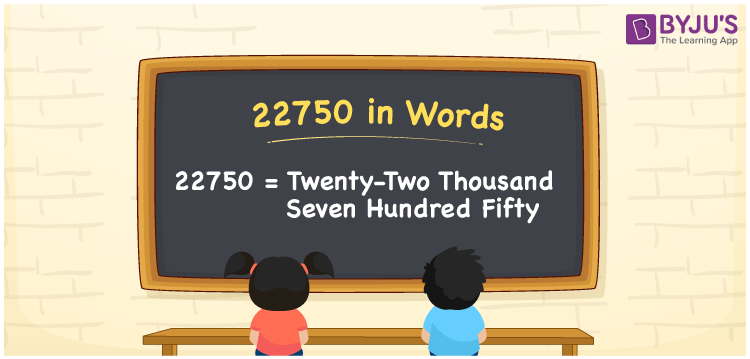# 22750 in Words

The number 22750 in words is Twenty-two thousand seven hundred fifty. For example, Ramya has purchased clothes for Rs. 22750. Hence, we can say that 22750 is a cardinal number. For writing the number in words, we generally employ the place value system. Here, we have discussed the method of writing the number 22750 in words in detail.

 22750 in Words: Twenty-two Thousand Seven Hundred Fifty. Twenty-two Thousand Seven Hundred Fifty in Numerical Form: 22750.

## 22750 in English Words## How to Write 22750 in Words?

As we know, the place value table is used to write the number names. The following are the place values of the number 22750.

 Ten-thousands Thousands Hundreds Tens Ones 2 2 7 5 0

The expanded form of 22750 is as follows:

= 2 × Ten thousand + 2 × Thousand + 7 × Hundred + 5 × Ten + 0 × One

= 2 × 10000 + 2 × 1000 + 7 × 100 + 5 × Ten + 0 × One

= 20000 + 2000 + 700 + 50

= 22750

= twenty-two thousand seven hundred fifty

Hence, 22750 in words is twenty-two thousand seven hundred fifty.

22750 in words – Twenty-two thousand seven hundred fifty

Is 22750 an odd number? – No

Is 22750 an even number? – Yes

Is 22750 a perfect square number? – No

Is 22750 a perfect cube number? – No

Is 22750 a prime number? – No

Is 22750 a composite number? – Yes

## Frequently Asked Questions on 22750 in Words

Q1

### How to spell 22750 in words?

22750 in words is twenty-two thousand seven hundred fifty.

Q2

### Simplify 22000 + 750, and express it in words.

Simplifying 22000 + 750, we get 22750. Hence, 22750 in words is twenty-two thousand seven hundred fifty.

Q3

### Is 22750 a prime number?

No, 22750 is not a prime number.